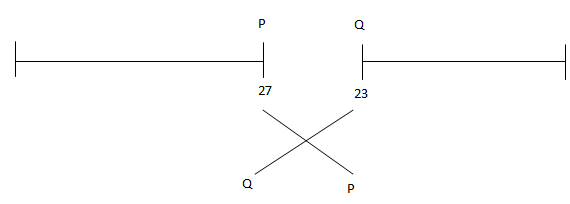# TARGET SBI EXAM 2018: REASONING –Order & ranking – 1

Dear Bankersdaily Aspirants,

Hope , Your preparation for SBI Clerk & PO exam 2018 is good . Since SBI Clerk & PO exam is a Two level which will be conducted in an online mode, Competition will be higher for this SBI Clerk & PO Exam and the most important thing , Current affairs section will be there in this exam. So aspirants have to concentrate in Four sections namely Aptitude, Reasoning, English, Current Affairs.

The person with high speed and very good accuracy only can crack this exam to taste the success. The candidates will be selected on the basis of performance in this online exam test. So the candidate with consistent hard work and regular practice will crack the exam very easily.

Here are some questions which will help you to identify your level of preparation.

Exam: SBI Clerk & PO Exam 2018

Topic: Order & ranking – 1

Timing: 7 minutes

Q.1) In a row of friends P and Q are stand in the 27th from the left and 23rd from the right end respectively. If the inter changed their position Q is 35th from the right end then how many persons sits between P and Q?

a) 13

b) 11

c) 17

d) 9

e) 12

D.2-4) Study the following information carefully and answer the given questions

Among the five friends A, B, C, D and E are has different weight. Only two person are lighter than D. E is lighter than D but heavier than C. The one who is the second heaviest among them is of 80kg.

Q.2) Which of the following statement is true?

a) A is heavier than B

b) A’s weight is more than 80kg

c) C is heavier than D

d) E’s weight is less than 80kg

e) None of these

Q.3) How many person heavier than B?

a) None

b) One

c) Two

d) Three

e) Can’t be determined

Q.4) If A’s weight is 93kg then who is second heaviest?

a) B

b) E

c) D

d) A

e) C

D.5-7) Study the following information carefully and answer the given questions

Among six friends P, Q, R, S, T and U each them is having different height. Only two persons are shorter than P. Q is shorter than P and S, but is not the shortest. R is tallest among them.

Q.5) Who is third shortest?

a) P

b) R

c) S

d) T

e) Q

Q.6) Who is shortest among them?

a) R

b) U

c) T

d) Q

e) Either U or T

Q.7) Who is fifth tallest among them?

a) P

b) R

c) Q

d) T

e) S

D.8-10) Study the following information carefully and answer the given questions

There are five students A, B, C, D and E each of them scored different marks in exam. A is scored less mark than B. D is scored less mark than C. C is scored more mark than A. Highest mark is 83%. A is Second lowest scored 47%.D is scored not lowest mark and score more than B.

Q.8) Who is scored highest mark?

a) D

b) B

c) C

d) A

e) None of these

Q.9) Who is scored third highest mark?

a) A

b) E

c) B

d) C

e) D

Q.10) What is possible of marks of E?

a) 75%

b) 50%

c) 65%

d) 40%

e) None of these

Answer Key

1. (b)27 + 35 – 1 = 61

61 – 50 = 11

D.2 – 4)

A/B

A / B = 80 kg

D

E

C

2. (d)

3. (e)

4. (a)

D.5-7)

R

T/V

P

S

Q

T/V

5. (a)

6. (e)

7. (c)

D.8-10)

C

D – 83%

B

A – 47%

E

8. (c)

9. (c)

10. (d)

You may also like:

TARGET SBI EXAM 2018: REASONING -Alphanumeric series